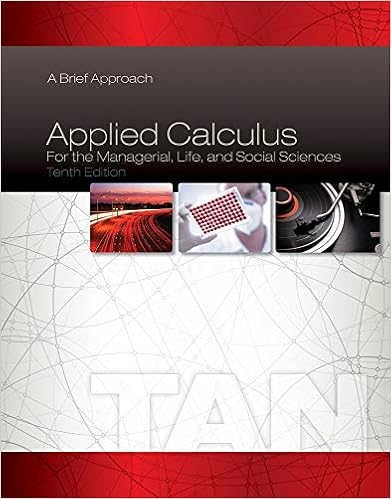# Problems_S2.4-2.5.pdf - MATH 140 Fall 2016 Problems from...

• Ammarnorain
• 1

This preview shows page 1 out of 1 page.

##### We have textbook solutions for you!
The document you are viewing contains questions related to this textbook.The document you are viewing contains questions related to this textbook.
Chapter 3 / Exercise 39
Applied Calculus for the Managerial, Life, and Social Sciences: A Brief Approach
TanExpert Verified
MATH 140, Fall 2016Problems from Sections 24 and 2.51.(Fa09)Let(u1D465) =u1D453(u1D454(u1D465)), whereu1D453andu1D454are differentiable functions. Finduni2032.var(4)givenu1D454(2) =13,u1D454(4)=1,u1D453uni2032.varparenleft.s213parenright.s2=12,u1D453uni2032.var(2)=−1,u1D454uni2032.var(2)=3andu1D454uni2032.var(4)=0.2.(Fa12)Suppose thatu1D454(3) = 2andu1D454uni2032.var(3) = 4. Find the slope of the tangent line to the graph of(u1D465) =u1D454(u1D465)u1D4652−4at the pointparenleft.s23,25parenright.s2.28(a)u1D45A=3225(b)3(c)u1D45A=32(d)25(e)u1D45A=8
3. (Fa14)Ifu1D453(u1D465)=11−7u1D465, findu1D453uni2032.varuni2032.var(0).
4. (Fa14)Find allu1D465-coordinates of all points on the graph of the functionu1D453(u1D465) = 2sin2u1D465−2cosu1D465in theintervalbracketleft.s2
##### We have textbook solutions for you!
The document you are viewing contains questions related to this textbook.The document you are viewing contains questions related to this textbook.
Chapter 3 / Exercise 39
Applied Calculus for the Managerial, Life, and Social Sciences: A Brief Approach
TanExpert Verified
•••